﻿ Ether, Dark Matter and Topology of the Universe

### Ether, Dark Matter and Topology of the Universe

Thierry DELORTOPEN ACCESSPEER-REVIEWED

## Ether, Dark Matter and Topology of the Universe

Thierry DELORTDepartment of Physics, Inria Saclays, Saclays, France

### Abstract

The article is divided in 2 parts. In the 1st part (PART I) we propose that a substance, called ether-substance, fills and constitutes all what is called “vacuum” in the Universe. We assume that it has a mass and consequently it could be the nature of dark matter. Modelling it as an ideal gas, we obtain the flat rotation curve of spiral galaxies. Using a very simple model of thermal transfer between baryonic particles and ether-substance, we obtain the baryonic Tully-Fisher’s law. So we introduce a new concept of ether, different from the pre-relativistic concept of ether, and we called “Cosmology based on ether” (CBE) Cosmology based on this new concept. In this CBE, topology of the Universe is much simpler and more attractive than topologies proposed by the Standard Cosmological model (SCM) (whose some fundamental aspects are kept in CBE). We propose 2 models in CBE. The first one does not need dark energy nor cosmological constant, and does not need the complex mathematics of General Relativity, contrary to SCM (and to the 2nd model of CBE). Nonetheless, we obtain in the 1st model of CBE a very simple Hubble’s constant, in 1/t, t age of the Universe, and many cosmological observations that were previously explained only by the SCM. Moreover we interpret in both models of CBE the Referential in which fossil radiation is isotropic. CBE is, as SCM, compatible with Special and General Relativity, despite that it is based on a new concept of ether. In the 2nd part, (Part II), we will study some problems raised by the Part I (motion of galaxies in the space, concentration of ether-substance around stars…).

### At a glance: Figures

1
Prev Next

• DELORT, Thierry. "Ether, Dark Matter and Topology of the Universe." International Journal of Physics 3.1 (2015): 17-28.
• DELORT, T. (2015). Ether, Dark Matter and Topology of the Universe. International Journal of Physics, 3(1), 17-28.
• DELORT, Thierry. "Ether, Dark Matter and Topology of the Universe." International Journal of Physics 3, no. 1 (2015): 17-28.

 Import into BibTeX Import into EndNote Import into RefMan Import into RefWorks

### 1. Part I

1.1. Introduction

After the theory of Special Relativity was admitted, the idea of ether has been abandoned in physics. Indeed it appeared that the existence of ether was not necessary in order to explain the results of experiments in general physics, in particular in particle physics and electromagnetism.

In this article, we will see that a new concept of ether, different from the old pre-relativistic concept of ether, appears to be fundamental in Cosmology, because it permits to interpret many observations that cannot be interpreted in the Standard Cosmological model [5, 6] (SCM), for instance the flat rotation curve of galaxies, the Tully-Fisher’s law, a simple topology of Universe, Referential in which fossil radiation is isotropic… Nonetheless we will see that this new Cosmology, called Cosmology based on Ether (CBE) interpret successfully all astronomical observations that were previously only interpreted by SCM, and moreover is compatible with Special and General Relativity.

The 3 fundamental following points, that were valid in SCM, remain valid in CBE:

1. The Universe is in expansion.

2. The factor of expansion 1+z interacts with the length of wave of photons and with intervals of distance between photons exactly the same way as in classical Cosmology.

3. The Big-Bang existed, and fossil radiation was emitted just after the Big-Bang.

We could have added in the point 2 that the factor of expansion 1+z is obtained using the equations of General Relativity in order to be closer to the SCM. We will do this in the second model of the CBE. But we will choose (1st model of the CBE) another solution that despite of its great simplicity brings to obtain predictions in agreement with all astronomical observations. (In particular a Hubble’s constant in 1/t, t age of the universe, and commoving, angular and luminosity distance in agreement with observation).

The CBE is based on 4 simple Hypothesis, A,B,C,D. The new concept of ether involves 2 different kinds of ether: We call the 1st one local ether, that is a kind of Referential, the 2nd one, called ether-substance, is a substance filling all Universe. We will see also later (Hypothesis Da) a 3rd kind of ether, called absolute ether, that is also a particular Referential. To begin with, we make 2 fundamental hypothesis defining partially according to CBE local ether and ether-substance:

A. a) At any point of the space, it exists a very particular local Referential, called local Ether, defining the local rest. Those local referentials define absolute time (indicated by clocks at rest in those referentials) that is the age of the Universe, and local distances (indicated by rules at rest in the local Referentials). If D is the local distance covered by a photon within an absolute time T, D=cT. (This means that locally the velocity of light relative to this local Ether is equal to c and we obviously admit that physical laws have their classical expressions expressed in the local Ether).

(We could consider that the existence of such a local ether contradicts Special Relativity (S.R) for which it does not exist a special frame of reference among all Galilean Referentials. In order to answer to this objection 2 remarks:

Firstly according to observation it exists a very particular Referential in which fossil radiation is isotropic. Such a Referential can obviously be considered as a special frame of reference and consequently the observation of this particular Referential, that is an experimental fact, contradicts itself Special Relativity. We will see that according to CBE, it is this particular Referential that is the local ether.

Secondly we will assume in CBE that this local ether, as all Galilean Referentials, are Lorentz Referentials (meaning that Lorentz transformations are valid between them). Consequently all the classical laws (in electromagnetism, particles physics..) can be applied in each Galilean Referential, in agreement with Special Relativity. Consequently none classical experiment linked to S.R (especially in electromagnetism, particles physics, but not including the experiment detecting the Referential in which fossil radiation is isotropic), can permit to detect the local ether

So we see that the 1st Principle of S.R “In each Galilean Referential RG, physical laws are valid” remains valid in CBE except for the physical law “ Fossil radiation is isotropic in RG” that can obviously be valid for at most one Galilean Referential, (at a given point P of the Universe ) and is moreover an experimental fact).

b) The velocity (in norm and vector, assuming that local ethers have parallel axes) relative to a local ether (called local velocity) of a photon (traveling in the Universe) keeps itself.

B. The vacuum is filled by a substance, called ether-substance. It owns a mass and can be modeled as an ideal gas.

(This ether-substance is only affected by gravitational interaction. So we cannot detect it using electromagnetic, weak and strong interaction. But we will see that we can detect it using gravitational interaction (flat rotation curve of galaxies).

We see that the points 1,2,3 of SCM, also admitted in CBE, are a priori compatible with the hypothesis A, B of the CBE. In fact those points 1,2,3 are consequences of hypothesis A, B, C, D.

In the first sections of the PART I we will only use the hypothesis B, introducing also an hypothesis C expressing a thermal transfer between baryons and ether-substance. We will obtain using those 2 hypothesis the flat rotation curve of galaxies and the Tully-Fisher’s law.

In the following sections of the PART I, we will use the hypothesis A, and we will introduce a new hypothesis D defining the topological form of the Universe (using also our concept of dark matter as ether-substance), and defining completely local ethers, at each point of the Universe. We will them interpret the observation of Cosmological distances, Hubble’s constant, fossil radiation, supernova explosion…

In order to interpret those observations in CBE, we will propose 2 models: The 2nd model of CBE is based on mathematics of General Relativity, as SCM. Consequently it should also require dark energy and Cosmological constant. The 1st model of CBE is based on very simple mathematics and does not need dark energy nor cosmological constant.

We will see that our theory appears to be an alternative to MOND theory [9, 10] in order to interpret phenomena linked to dark matter. Naturally we define locally Galilean Referentials as Referentials with axes parallel to the axes of the local ether, and driven with a constant velocity relative to it. Then the hypothesis A and B are compatible with Special Relativity: To justify this, we just need to assume that the local Ether is a Lorentz Referential, as all Galilean Referentials. But we remind that the existence of a very particular Referential in which fossil radiation is isotropic, is an experimental fact. And it will be this Referential that will be identified with the local Ether defined in point A. The hypothesis A,B,C,D are also compatible with General Relativity.

(It is also possible to build a general physical theory in which the local ether is not a Lorentz Referential as all Galilean Referentials, that is compatible with all experiments connected to Special Relativity. This theory has been exposed in the references [2, 3], but is not necessary for the presently exposed theory. It has nonetheless the advantage to interpret the quantum experiments in which an information is translated faster than the velocity of light .)

We are going to show how those hypothesis A and B permit to solve enigmas connected to dark matter and to fossil radiation.

This article can also be read in . It is possible that some future improved version be put on the same website.

1.2. Dark Matter
1.2.1. Nature of Dark Matter-Its Invisibility

If we admit that the ether-substance has a mass, then it is clear that dark matter could be constituted of ether-substance. So this gives the nature of dark matter and the origin of its invisibility, because it constitutes what we call the vacuum and consequently it is obviously transparent.

1.2.2. Curves of Velocity of Stars in Galaxies

If we model the ether-substance as an ideal gas, and if we consider that galaxies are concentrations of ether-substance, we obtain that the velocity of stars is independent of their distance to the center, this constituting an enigma of classical Cosmological standard model.

So we make the following hypothesis that the ether-substance can be modeled as an ideal gas:

An element of Ether-substance with a mass m, a volume V, a pressure P and a temperature T verifies the law, k0 being a constant: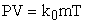(1)

Which means, setting k1=k0T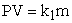(2)

Or equivalently, ρ being the density of the element: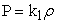(3a)

We then emitted the hypothesis that a galaxy could be modeled as a concentration of Ether-substance presenting a spherical symmetry, at a constant and homogeneous temperature T.

We then considered the sphere S(r) (resp.the sphere S(r+dr)) that is the sphere inside the concentration of Ether-substance with a radius r (resp. r+dr) and whose the center is the center O of the galaxy. S(O,r) is the full sphere of radius r and of center O.

The mass M(r) of the full sphere is given by: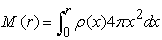(3b)

We then consider the following equation (4) of equilibrium of forces on an element of Ether-substance with a surface dS, a width dr, situated between the 2 spheres S(O,r) and S(r+dr):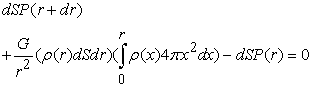(4)

Eliminating dS, we obtain the equation: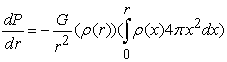(5)

And using the equation (3), we obtain the equation: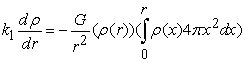(6)

We then verify that the density of the ether-substance ρ(r) satisfying the preceding equation of equilibrium is: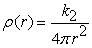(7)

(A density of dark matter expressed as in Equation (7) has already been proposed in order to explain the flat rotation curve of spiral galaxies, but here not only we justify the invisibility of this dark matter, but also we give a theoretical justification of this expression, consequence of the model of ether-substance as an ideal gas, Equation (1)).

The constant k2 being given by, G being the Universal attraction gravitational constant: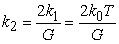(8)

Using the preceding equation (7), we obtain that the mass M(r) of the sphere S(O,r) constituted of Ether-substance is given by the equation: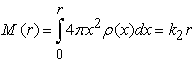(9)

We then obtain, neglecting the mass of stars in the galaxy, that the velocity v(r) of a star of a galaxy situated at a distance r from the center O of the galaxy is given by v(r)2/r=GM(r)/r2 and consequently: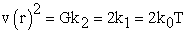(10)

So we obtain in the previous equation (10) that the velocity of a star in a galaxy is independent of its distance to the center O of the galaxy, solving the 3rd enigma concerning dark matter. (We previously solved the enigma of the nature of dark matter and of its invisibility).

We note that the theoretical elements of the new Cosmology permitting to obtain the equations (7)(8)(9)(10) are compatible with Special and General Relativity Principles.

1.2.3. Tully-Fisher’s Law.
1.2.3.1. Recall

We remind that the Tully-Fisher’s law is the following:

Tully and Fisher realized some observations on spiral galaxies. They obtain that the luminosity L of a spiral galaxy is proportional to the 4th power of the velocity v of stars in this galaxy. So we have the Tully-Fisher’s law for spiral galaxies, K1 being a constant: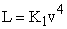(11)

But the baryonic mass M of a spiral galaxy is usually proportional to its luminosity. So we have also the law for a spiral galaxy, K2 being a constant: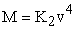(12)

This 2nd form of Tully-Fisher’s law is known as the baryonic Tully-Fisher’s law.

We remind that the Tully-Fisher’s law (11) is not verified in general for galaxies that are not spiral galaxies. But the observations of Mc Gaugh  show that the baryonic Tully-Fisher’s law (12) seems to be true for all galaxies. This constitutes a new major enigma for the classical Cosmology, but we are going to see how we can derivate this law from the existence of ether-substance.

1.2.3.2. Theory of Quantified Loss of Calorific Energy (by Nuclei)

We saw in the previous equation (10) that according to the new Cosmology, the square of the velocity of stars in a galaxy is proportional to the temperature of the concentration of Ether-substance constituting this galaxy. So if we determine this temperature T, we then obtain the squared velocity of the stars in this galaxy. So we need to try to determine T:

-A first possible idea is that the temperature T is the so called “Temperature of the fossil radiation”. But this is impossible because it would imply that all stars of all galaxies are driven with the same velocity and we know that it is not the case.

-A second possible idea is that the temperature T is due to the absorption by the concentration of Ether-substance constituting the galaxy of a fraction of the photons emitted by the stars of this galaxy. But if it was the case, the temperature and consequently the velocity of the stars of the galaxy would only depend on the luminosity of the galaxy, and we should have a law analogous to the Law of Tully-Fisher (11) and we know that it is not the case.

-A third possible idea is that in any galaxy, each baryon interacts with the Ether-substance constituting the galaxy, and consequently it occurs for each baryon a loss of calorific energy communicated to the Ether-substance.

A priori we could expect that this loss of calorific energy for each baryon (transmitted to the Ether-substance) depends on the temperature of this baryon, but if it was the case, the total calorific loss for all baryons would be extremely difficult to calculate and moreover we would not obtain that the total calorific loss depend on the baryonic mass of the galaxy.

The final idea is that indeed it occurs a calorific loss for each atom (transmitted to the Ether-substance), but that this loss is quantified, depending only on the number of the nucleons of the nucleus of the atom. This loss should be very low, but the calorific capacity of the Ether-substance being also very low, it can involve an appreciable temperature of the concentration of Ether- substance constituting the galaxy.

So we make the following Hypothesis Ca):

-Each nucleus of atom in a galaxy is submitted to a loss of calorific energy, transmitted to the concentration of Ether-substance constituting the galaxy.

-This loss of calorific energy depends only on the number of nucleons constituting the nucleus (It is independent of its temperature). So if p is the power corresponding to the loss of calorific energy for a nucleus of atom with n nucleons, it exists a constant p0 (loss of calorific energy per nucleon) such that: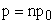(13)

According to the equation (13), the total power corresponding to the loss of calorific energy by all the atoms in a galaxy is proportional to the number of nucleons of the whole of those atoms, and consequently to the baryonic mass of this galaxy. So if m0 is the mass of one nucleon, M being the baryonic mass of the galaxy, we obtain according to the equation (13) that the total power Pr corresponding to the calorific energy received by the concentration of Ether-substance constituting the galaxy from all the atoms is given by the following equation, K3 being the constant p0/m0: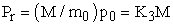(14)

Concerning the preceding hypothesis Ca) it is important to remark:

-The loss of calorific energy of a baryon transmitted to the Ether-substance is a quantum phenomenon, consequently it is not surprising that the power corresponding to the loss of calorific energy of a baryon be quantified.

-In physics of thermal transfer, the calorific loss of one or several particles usually depends on their temperature. But it is always only thermal transfers from atoms towards other atoms that are considered, and consequently it is not compulsory that it be also the case for transfers between atoms and Ether-substance.

-It is possible that this hypothesis be true only for atoms whose temperature be superior to a given temperature TS. Moreover, it is also probable, but not certain, that their temperature must be superior to the local temperature of the Ether-substance.

-The great simplicity of this hypothesis permits to obtain very easily the total power corresponding to calorific energy received by the concentration of Ether-substance (Equation (14)). If the loss of energy of a nucleus of atom depended on its temperature, then it would be incomparably more complicated, and maybe impossible, to obtain a simple expression giving this total power.

-This hypothesis is a priori compatible with the Special and General Relativity Principles, and also with classical Quantum Physics.

1.2.3.3. Obtainment of the Baryonic Tully-Fisher’s Law

In agreement with the previous model of galaxy, we model a galaxy as a concentration of Ether-substance presenting a spherical symmetry (and consequently being itself a sphere), at a temperature T and immerged inside a medium constituted of Ether-substance at a temperature T0 and with a density ρ0.

In order to obtain the radius R of the concentration of Ether-substance constituting the galaxy, it is logical to make the hypothesis of the continuity of ρ(r): R is the radius for which the density ρ(r) of the concentration of Ether-substance is equal to ρ0. So we have the equation: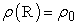(15)

Consequently we have according to the equations (7) and (8):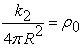(16)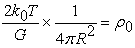(17)

So we obtain that the radius R of the concentration of Ether-substance constituting the galaxy is given approximately by the equation: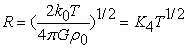(18)

The constant K4 being given by: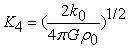(19)

We can then consider that the sphere with a radius R of Ether-substance constituting the galaxy is in thermal interaction with the medium at a temperature T0 in which it is immerged. We make the hypothesis C)b):

The thermal interaction between ether-substance constituting the galaxy and surrounding intergalactic ether-substance is a convection phenomenon.

We know that if φ is the thermal flow of energy on the borders of the sphere, the power Pl lost by the sphere of Ether-substance constituting the galaxy is given by the equation: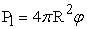(20)

But we know that for a convection phenomenon between a medium at a temperature T and a medium at a temperature T0 the flow φ between the 2 media is classically given by the expression, h being a constant depending only on ρ0: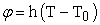(21)

Consequently the total power lost by the concentration of Eher-substance is: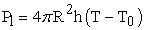(22)

We can consider that at the equilibrium, the thermal power Pr received by the concentration of Ether-substance constituting the galaxy is equal to the thermal power Pl lost by this concentration. Consequently according to the equations (14) and (22), M being the baryonic mass of the galaxy, we have: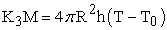(23)

Using then the equation (18):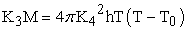(24)

Making the approximation T0<<T: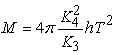(25)

Consequently we obtain the expression of T, defining the constant K5: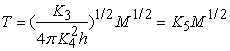(26)

And then according to the equation (10):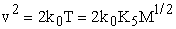(27)

So: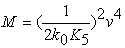(28a)

So we finally obtain: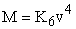(28b)

The constant K6 being defined by: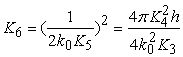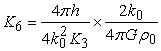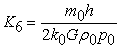(28c)

So we obtain the baryonic Tully-Fisher’s law (12), with K2=K6. It is natural to assume that h depends on ρ0. The simplest expression of h is h=Cρ0, C being a constant. With this relation, K6 is independent of ρ0, and we can use the baryonic Tully-Fisher’s law in order to define candles used to evaluate distances in the Universe.

1.2.4. Temperature of the Ether-substance

So we saw that in our interpretation of dark matter, according to the equation (10), the temperature of the ether-substance constituting a galaxy is proportional to the squared velocity of the stars in this galaxy.

We have seen that this temperature could not be the temperature of fossil radiation, because it would then imply that the velocity be always the same.

We could also suppose that this temperature is superior to the temperature of fossil radiation, considering that this temperature of fossil radiation is the temperature T0 used in equation (21), (temperature of intergalactic ether-substance) but then we find a new problem:

According to observation, the velocities of stars for different galaxies can vary with a factor 10. This implies that the temperature of galaxies vary with a factor 100. Consequently if in the equation (21) T0 was the temperature of fossil radiation (2,73 °K), the temperature of some galaxies should be more than 3000°K, which seems to be very improbable.

So we have the possible explanations:

a) The temperature T0 in equation (21) is far less than the temperature of fossil radiation.

b) Baryons can emit thermal power towards ether-substance even if their temperature is inferior to the temperature of ether-substance, and thermal transfer from ether-substance towards baryons in nil or negligible.

The previous explanation a) is possible considering that the ether-substance does not interact with fossil radiation.

1.2.5. Form of the Universe

If the Universe was completely isotropic, we could expect by symmetry that the thermal flow inside the ether-substance through a great surface be nil. Consequently the temperature of the ether-substance inside a great sphere of the Universe (For instance with a radius of 5 billion years) should increase and tend to a uniform temperature of the ether-substance inside the sphere. We know that it is not the case because galaxies have not the same temperature and moreover we admitted that the temperature of the intergalactic ether-substance is by far inferior to the temperature of the ether-substance inside galaxies. So an infinite Universe would contradict the observation.

With our model of dark matter, ether-substance constituting what is called “vacuum”, it is very easy to define a spherical Universe: This Universe is a sphere of ether-substance, (in which galaxies are immerged), surrounded by another medium called “nothingness”, that is not ether-substance. It was not possible to define such a spherical Universe in the SCM: Only surfacic models of the Universe, as plane, spherical or in horse’s saddle were possible in the SCM. Moreover, a spherical model as the one we assume in CBE, is in contradiction with the 1st Cosmological Principle of the SCM, expressing that the Universe is isotropic observed by any point of the Universe. Nonetheless, we remark that in CBE, the spherical Universe should be isotropic observed from its center, or from a point sufficiently far from its borders.

And in the case in which the Universe is a sphere, we avoid the previous contradiction. Indeed we can consider that in the borders of the Universe (supposed to be spherical), there is a phenomenon of thermal convection: T being the temperature of the intergalactic ether-substance, supposed to be uniform, we can consider that there is a convective thermal transfer between a medium at a temperature T and a medium at a temperature T0=0. Then the expression of the thermal flow lost by the Universe at its borders is, k being a variable or a constant: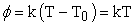(28d)

M being the baryonic mass of the Universe, we obtain from equation (14) that the equation of thermal equilibrium at the borders of the universe is: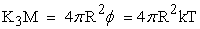(29a)

So we see that if the Universe increases from a factor f, according to the Equation (29a), if k is a constant, the temperature T of the intergalactic ether-substance diminishes from a factor f2. Here we supposed that k is independent of the density of the intergalactic ether-substance. If we had supposed that k=C2ρ0, ρ0 being the mass density of the intergalactic ether-substance and C2 being a constant, it is very easy to obtain that if the Universe increases from a factor f, then T also increases by a factor f which is impossible.

We remark that our convective thermal model is valid even if the galaxy is at rest relative to the local “sea” of ether substance in which it is immerged. Indeed elements of the ether substance constituting the concentration of the ether substance in a galaxy are not dissolved in the “sea” of ether substance surrounding the galaxy. So we have a convective thermal transfer.

So we see how our model of dark matter brings us to obtain an Universe that is not completely isotropic. Nonetheless, it is logical to assume that it is isotropic observed from the center of the spherical Universe, admitting that the Universe presents a spherical symmetry.

1.2.6. Topology of the Universe-Law of Hubble-redshift (1st model)

If we consider a photon emitted from a point A at an absolute time tA (We remind that t is the age of the Universe) and arriving at a point B at a time tB, then we will call time-back distance the sum of elementary local distance covered by the photon between tA and tB D=c(tB-tA).

Indeed according to the hypothesis A, we know that if the photon covers an elementary local distance dD within an interval of absolute time dt, we have dD=cdt. If we sum all those elementary distances and absolute intervals of time we obtain D=c(tB-tA).

We consider the simple model of a Universe as a swelling sphere. Such a model with borders moving at a constant velocity c and the absolute velocity of light being equal to c relative to an absolute Referential is completely described in [3, 4]. But in the models exposed in this present article (1st and 2nd models of the CBE), borders of the Universe do not move at the constant velocity c and the velocity of light is equal to c relative to a local Referential.

So we make the 4th hypothesis Da,b:

Da)The universe is like a swelling sphere whose borders move either (1st model of CBE) at a constant absolute velocity C (relative to a Referential, called absolute ether, whose the origin is in O center of the swelling sphere), or (2nd model of CBE) in agreement with the equations of General Relativity, relative to the absolute ether.

We define commoving points of the swelling sphere the following way:

P(t) is any point belonging to the border of the swelling sphere, with OP(t) remaining in the same direction u, fixed vector of the absolute ether. ( We have in the 1st model of CBE OP(t)=Ct).

A commoving point A(t) of the swelling sphere is defined by :

-A(t) is in the segment [O,P(t)]

-OA(t)=aOP(t), a being a constant belonging to [0,1][, 1].

So in particular O and P(t) are commoving points of the swelling sphere. Moreover if A(t) and B(t) are 2 commoving points of the swelling sphere, belonging both to a radius [O,P(t)], and if t1 and t2 are 2 ages of the Universe, f being the factor of expansion of the Universe between t1 and t2 (By definition f=OP(t2)/OP(t1)), then we have the 2 relations: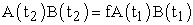And: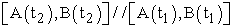Using Thales theorem we obtain the 2 previous relation A(t) and B(t) being any commoving points of the swelling sphere (not compulsory belonging both to the same radius [O,P(t)]).

We remark that in the 1st model, if A(t) is a commoving point of the swelling sphere, with preceding notations OA(t)=aCt, consequently the absolute velocity of A(t) is constant.

We naturally admit (Hypothesis Db) that commoving points of the swelling sphere are origins of the local ethers (See hypothesis A in 1.1. Introduction).

So according to the 1st model of CBE, the Universe is like a swelling sphere whose borders move at the absolute velocity C. This model is very simple but despite of its simplicity it is in agreement with all Cosmological observations. Moreover, it will help a lot to understand the 2nd model. We remind that defining an absolute Referential whose the origin is fixed in the point O center of the spherical Universe, this Referential defines “absolute distances” and “absolute velocities”. The time of this Referential being the time of the local ethers, we will call “absolute ether” this absolute Referential. So the velocity C of points belonging to the surface of the swelling sphere is measured in the absolute ether. In the 1st model CBE, at a commoving point of the swelling sphere A(t) of a radius [O,P(t)] of the spherical Universe with OA(t)=aOP(t) (OA(t) and OP(t) absolute distances), the local ether in A(t) is driven with a velocity vA=aC in the direction OP. So this velocity vA is constant.

Let us suppose the validity of the 1st model of CBE and that from a point O (O can be the center of the Universe, but not compulsory) at the present age of the Universe t0, we observe a photon coming from a point Q situated at a time-back distance D of O. We know according to the definition of time-back distance that a photon coming from Q and arriving at O at the time t0 was emitted at an absolute time tQ=t0-D/c. We know that at the time tQ the radius of the Universe was equal to CtQ and at the time t0 it was equal to Ct0. Consequently the factor of expansion of the Universe between tQ and t0 is (according to the 1st model of CBE):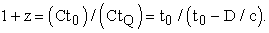(29b)

When D/ct0<<1 we obtain z=D/ct0 and consequently the Hubble’s constant is equal to 1/t0. The above equation is very simple and can easily be verified. For instance taking t0=15 billion years, we know that for z=0.5, D=5 billion light years and we have 1+z=t0/(t0-D/c). For z=9 we obtain D=13.5 billion years.

It is important to remark that D is not the luminosity distance, but the time-back distance that we defined as the distance that is the sum of elementary local distance covered by a photon.

We can define a luminosity distance, a commoving distance, an angular diameter distance that are completely analogous to those distances in classical Cosmology.

For instance let us suppose that we are at time t0 in the center O of the Universe, and we observe a photon emitted by a galaxy with a redshift z0. We suppose that this galaxy (observed with the redshift z0) was at a commoving point A(tE) of the swelling sphere, when the photon was emitted at the age tE of the Universe.

Then according to our definition of the time-back distance, the time- back distance of A is (observed at t0 in O):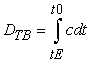(29c)

With dt is the absolute time taken by the photon (emitted by the galaxy) between the time t and t+dt.

z being any redshift between z0 and 0, we know that the photon covers the local distance cdt during the absolute time dt. We know that this distance is increased by the factor 1+z when the photon has reached O at time t0. Consequently the absolute distance between A(t0) (position of A(t) at time t0) and O is by definition the commoving distance: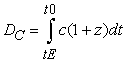(29d)

And according to Equation (29b) 1+z=t0/t. Consequently DC=ct0Log(1+z0).

From this expression of DC we can easily define the luminosity distance DL and the angular distance DA: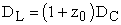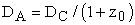(29e)

We remark that in our definition, there are the same relations between DTB, DC, DL, DA as in classical Cosmology.

We took an age of the Universe approximately equal to 15 billion years corresponding to a Hubble’s constant H=1/t0 approximately equal to 65 km/sMpc-1 despite that it is generally admitted that the Hubble’s constant H is approximately equal to 72km/sMpc-1 corresponding to a time t=1/H approximately equal to 13,5 billion years.

But many astrophysicists disagree with a Hubble’s constant approximately equal to 72 km/s Mpc-1 and find a Hubble’s constant approximately equal to 65km/sMpc-1. For instance Tammann and Reindl  in a very recent article (October 2012), after having reminded that astrophysicists disagree concerning the estimation of Hubble’s constant, then give a possible origin of the mistake leading to obtain a Hubble’s constant approximately equal to 72 km/s Mpc-1, and finally find themselves a Hubble’s constant of 63,7 (+- 2.3) km/sMpc-1.

There is also a second possibility: Time-back distance could be superior to those predicted by the SCM by a factor of 5% to 7%. We cannot exclude this possibility.

We can interpret in CBE the observation of a supernova explosion [5, 11] the same way as in SCM: Indeed we have also in CBE (see point 2 of 1.INTRODUCTION, that is consequence of hypothesis A,B,C,D), that if the Universe is submitted to a factor of expansion f=1+z, then lengths of wave of photons and distance between 2 photons moving on the same axis increase also by a factor 1+z.

So we see that the 1st model of the CBE is in agreement with most Cosmological observations, despite that it does not need dark energy nor Cosmological constant nor the complex mathematics of General Relativity.

1.2.7. Evaluation of the Constant C, Velocity of the Borders of the Universe. (1st model)

It is important to remark that according to the 1st model, C is not a priori equal to c. But we may expect that C is strictly superior to c but is of the order of c (We will see that it is superior to 2c or 3c).

The fact that we observe from our galaxy an isotropic Universe indicates that our galaxy should be close to the center O of the spherical Universe, or at a great distance from its borders. We remark that the Universe could be homogeneous but not compulsory. It could explain why we do not observe traces of quasars and blue dwarfs in the neighborhood of our galaxy. Nonetheless the Universe should be isotropic observed from its center.

In the 1st model of the CBE, we can obtain an estimation of C, absolute velocity of the borders of the Universe, the following way:

We suppose that at time tE, a photon is emitted from the borders of the Universe towards the center O of the spherical Universe. We call x(t) the distance at t age of the Universe between O and the photon. According to our model, if v(t) is the velocity of the point of Ether-substance coinciding with the photon at an age of the Universe t, we have the relation: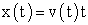(29f)

Indeed, we obtain immediately that if A(t) is a commoving point of the Universe, if x(t) is its distance to O and v is its absolute velocity, (we remind that it is constant), we have x(t)=OA(t)=vt. In the previous equation, we wrote v(t) because A(t) is the commoving point reach by the photon at a time t, age of the Universe.

Moreover, according with our model, remarking that the absolute velocity of the photon at time t is equal to v(t)-c: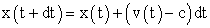(29g)

So we obtain successively: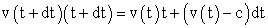(29h)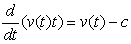(29i)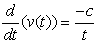(29j)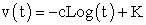(29k)

With the initial condition v(tE)=C, we obtain the constant K and we finally get: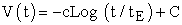(29l)

Consequently, the photon reaches O (with v(t0)=0) at time t0 with: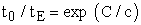(29m)

tE represents the lowest age of the Universe that we can observe at the present age of the Universe t0. Indeed, it is evident that if a photon is emitted from a galaxy at a time inferior to tE, it will reach O before t0, and consequently will be not observed at t0. We can also obtain the same result using the commoving distance.

In order to get C, we have also the equations, z0 being the greater redshift that we can observe (corresponding to an age of the Universe tE):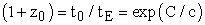(29n)

Moreover, we know that the greatest commoving distance D0 that we can observe at time t0 meaning the absolute distance between O and the borders of the Universe at time t0 is related with C by: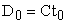(29o)

Presently, we know that the greatest commoving distance that we can observe is approximately D1=35 billion years, and the greatest observed redshift is z1=10.

We are sure that z0>z1, C=cLog(1+z0)>cLog(1+z1) which gives C>2,3c. If we admit that D0 is of the same order as D1 (which is possible), we obtain that C is of the order of 3. It is very possible that C be much greater than 2,3, for instance C=10. In that case, we could possibly observe the Universe when its age was only 1 million years

If photons were absorbed by the borders of the spherical Universe, it could be possible that presently we could not observe fossil radiation. Consequently we admit that photons simply recoil when they reach the borders of the universe.

We admit the following law of reflection :If the trajectory of a photon is along a radius of the spherical Universe, when the photon recoils against the internal surface of the spherical Universe, if its initial local velocity was c, it becomes –c. Consequently the photon remains on the same radius. We remark that we obtain easily that if a photon has a local velocity at is along a radius of the spherical Universe, then the photon remains along this radius (we remind, Hypothesis A, that the local velocity of a photon keeps itself).

Proceeding as in SCM, we obtain in CBE that if at time tF.R there are in all the Universe photons distributed in agreement with the radiation of a black body at a temperature TF.R, then at any time t greater than tF.R, there are also in all the Universe photons distributed in agreement with the radiation of a black body at a temperature T=TF.R /f, f=1+z being the factor of expansion between tF.R and t. This is a consequence of point 2 of introduction, that is itself consequence of hypothesis A,B,C,D. Consequently CBE is in agreement with the observation of the temperature of fossil radiation corresponding to a great 1+z . Also we interpret in CBE heterogeneities of fossil radiation the same way as in SCM. But in CBE, contrary to SCM, we can interpret very naturally the Referential in which fossil radiation is isotropic: It is the local ether.

In the case in which an observer is situated at a commoving point O’, we then consider the Referential R’ whose the origin is O’ and whose the axis are parallel to the axis of the absolute Ether R that we defined previously. We then can keep the same local Referentials that are analogous to local ethers that we defined for R (With commoving points as origin). We then remark that R’ behaves exactly as R: We generalize the law of reflection: If the trajectory of a photon is along the radius of a sphere whose the center is O’, when it reaches the borders of the Universe, if its initial local velocity was c, it becomes –c. (Indeed we obtain easily that local velocity of photons are the same in R as in R’). So we also obtain that the photon remains on the same radius after the recoil. Also, fossil radiation is also isotropic relative to local ethers.

Because photons recoil against the borders of the Universe, we should expect to be able to observe the images of galaxies reflected by the borders of the Universe. But in order to explain why we do not observe the images of reflected galaxies we have 3 possible explanations:

Let t0 be the present age of the Universe, tE the earliest age of the Universe that we can observe at time t0 in the center O of the spherical Universe (see the previous section 2.7), tG the time in which appeared the first galaxies, and tD the dark age in which the Universe was not transparent to the light of galaxies. We know that tD is of the order of 1 billion years and if we admit that oldest galaxies are 13.5 billions years old tG is of the order of 1.5 billion years (taking t0 =15 billion years)

It is easy to obtain that if tG>tE or tE<tD then we cannot observe the image of galaxies reflected by the borders of the Universe. Because in both case we obtain that the images of reflected galaxies arrive in O center of the spherical Universe after t0. For instance we can have tE=1 billion year and tG=1.5 billion years, or tE=1.5 billion years and tD=2 billion years.

We know that it is admitted that at the dark age, the light emitted by galaxies was absorbed despite that the fossil radiation was not absorbed. A third possible explanation would be that it is also the case for the borders of the Universe, that would reflect fossil radiation but not light of galaxies.

1.2.9. 2nd Model of CBE

So we saw that the 1st model of the swelling balloon, despite of its simplicity, is compatible with most of Cosmological observations.

Nonetheless, according to the equation (29d), with t0/t=1+z, the commoving distance between O center of the Universe and a point with a redshift z is DC=ct0Log(1+z). Consequently with z=10, DC is between 30 and 35 billion years (depending on the value of the age of the Universe), and we know that the true value is approximately 34 billion years. So we see that the 1st model is apparently in agreement with every classical astronomical observation.

Nonetheless, we can propose a 2nd model compatible with the classical equations of General Relativity used in classical Cosmology. So in our 2nd model, we keep the same topological form of the Universe (swelling balloon) and also the classical equations of General Relativity used in classical Cosmology, that are used to get the factor of expansion 1+z.

Consequently we have the equation, 1+z(t1,t2) factor of expansion of the Universe between the ages t1 and t2: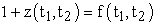(29q)

So f(t1, t2) is obtained using the equations of general Relativity. (In the 1st model,f(t1,t2)=t2/t1).

Then if R(t1) is the radius of the Universe at time t1, and t2>t1, the expression of the radius R(t2) is: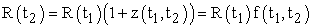(29r)

So we obtain that at time t2 the velocity of the borders of the Universe is (t1 being a constant):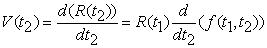(29s)

As for the 1st model, we remind that we defined a commoving point of the swelling sphere A(t) as point of a radius [O,P(t)] of the spherical Universe, P(t) point belonging to the border of the swelling sphere. A(t) is the origin of a local Ether and we have the relation, a being a constant: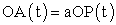(29t)

Moreover, as a consequence of the previous equation, if f is the factor of expansion of the Universe between t1 and t2: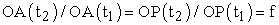(29u)

Consequently, the absolute velocity of A(t) (and consequently of the local ether whose the origin is in A(t)) is, if V(t) is the absolute velocity of P(t):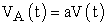(29v)

(We remind that as for the 1st model, we define an absolute Referential whose the origin is O center of the Universe. Absolute velocities are velocities measured in this absolute Referential).

In order to obtain the largest redshift that could be theoretically be observed, or equivalently the earliest age of the Universe that could be theoretically be observed, we proceed in a way completely analogously as for the 1st model:

We consider a photon emitted at time tE from a point P(tE) situated on the border of the Universe in the direction of O center of the Universe. We suppose that at time t the photon coincides with the point A(t) with OA(t)=a(t)OP(t). So we get the equations: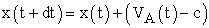(29w)

Or equivalently: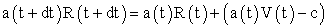(29x)

We see that this previous equation is analogous to the equation (29h).

In the 2nd model, fossil radiation is interpreted completely the same way as for the 1st model, photons recoiling on the borders of the Universe in the direction of their arrival.

The enigmatic dark energy used in classical Cosmology to justify the obtainment of the factor of expansion (equation (29q), could be the thermodynamic energy of the ether-substance, that we modeled as an ideal gas. We remind that dark energy is not necessary in the 1st model.

We remark that in our both models Universe is isotropic only observed from the center of the Universe o from commoving points situated at a distance sufficiently great from the borders of the Universe, contrary to the models of classical Cosmology in which it is isotropic observed from any point. Moreover, in our 2 models of CBE, the Universe is flat, and apparently does not need the phenomenon of inflation. The concept of ether-substance is important in order to justify the spherical form of the Universe. Indeed if the space was not filled by a substance it would not have been possible to delimit the borders of the Universe: In our both models borders of the Universe constitute the limit between the ether-substance and the “nothingless”).

We see that our both models contradict the famous Cosmological Principle because the Universe is not isotropic observed from most of the points of the Universe (except from the center O). We remind also that according to our both model, the space is not compulsory homogenous. This could explain why there were more quasars in the past and more blue galaxies: It is possible according to our both models that quasars and blue galaxies were more numerous closer to the borders of the Universe. As a consequence, they appear to be more numerous in the past.

1.3. Local Ether and Isotropy of Fossil Radiation

We know that fossil radiation is quasi isotropic in a Referential that is not interpreted in classical Cosmology. If an particular Referential (local ether) exists (Hypothesis A), then it is natural to assume that it is the Referential in which fossil radiation is quasi isotropic.

More precisely we know that in classical Cosmology we have the following fluctuations of temperature: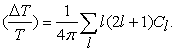(30)

In the previous expression l=1 is the dipole contribution, corresponding to the motion of our Referential linked to the earth relative to a particular Referential. Considering that this particular Referential is the local ether defines completely this Referential, that has none particular meaning in the classical Cosmology.

We also remark that if we consider the law:

“The fossil radiation is isotropic in the Referential R”,

we know that this physical law is true for only one Referential, which contradicts the Principle of Special Relativity and is in agreement with the hypothesis A of the existence of Ether.

1.4. Conclusion

So we see that the existence of local ethers and ether-substance as defined in the hypothesis A, B, C,D appears to be fundamental in order to interpret fossil radiation, the dark matter and the form of the Universe. It is very remarkable that the 3 admitted fundamental points 1,2,3 of CBE not only are compatible with the SCM, but also are consequences of hypothesis A,B,C,D. It is also remarkable that CBE, despite that it is based on the concept of ether, is compatible with Special Relativity, except concerning the fact that it exists a particular Referential in which fossil radiation is isotropic. But this last point is an experimental fact. In our 2nd model, we also have seen that the enigmatic dark energy could be the thermodynamic energy of the ether-substance. Our theory appears to be an alternative to MOND theory in order to interpret the flat rotation curve of galaxies. Contrary to the SCM, in the CBE Universe is not isotropic observed from any point, but it is isotropic observed from the center O of the Universe, or from a point sufficiently far from the borders of the Universe, and we assumed that it was the case for our galaxy.

We remark that in our 1st model of CBE, we obtained Hubble’s constant and the expression of the redshift due to expansion of the Universe in a new way without using the equations of general relativity. We also interpreted the nature of dark matter, the origin of its invisibility, the flat rotation curve galaxies, the baryonic Tully-Fisher’s law and the Referential is which fossil radiation is isotropic. We also proposed that the universe has a spherical form.

### 2. Part II

In PART I of this article, we presented a very complete cosmological theory of ether. In PART II we bring complements to this cosmology. In particular we study fluid mechanics for a spherical concentration of ether-substance moving inside the intergalactic ether-substance. We make also appear 2 kinds of radius for a galaxy and we establish the evolution as a function of time of the 1st kind. We also verify a simple relation between the 2 radius of the milky way at an age of the universe equal to 5 billion years. We also study the thermal transfer linked to the motion of a galaxy inside the intergalactic ether-substance (ether-substance occupying the space between galaxies).

2.1. Introduction

2.2. Motion of a Galaxy Inside the Intergalactic Ether-Substance

We could think that a spherical concentration of ether-substance such as a galaxy (or close to a star) moving inside the intergalactic ether-substance should be slowed down or be modified in mass because of the ether-substance surrounding this concentration.

In fact, we have 2 phenomena that we are going to justify further:

A. The moving spherical concentration of ether-substance keeps its mass.

B. The moving spherical concentration of ether-substance keeps its velocity: It is not slowed down nor accelerated.

Indeed, let us consider a spherical concentration of ether-substance (center O) driven with a velocity V relative to the local intergalactic ether-substance. Let us consider S, surface of a disk with the same radius as the sphere having its center in O, the plane of the disc being perpendicular to V.

Then in an interval of time dt, we have the 2 phenomena:

C. A volume SVdt of ether-substance is absorbed by the spherical concentration.(In front of the sphere).

D. A volume SVdt is emitted by the spherical concentration (to the back of the sphere).

Moreover we remark that the emitted and the absorbed volume of ether-substance have the same velocity and the same density.

Then the points A and B appear to be consequences of the points C and D and of the last remark.

2.3. Baryonic and Ethered Radius of a Galaxy

We know that the galaxy Andromeda is approximately at 2.5 billions year-light of our galaxy the milky way. We consider for instance the case of the milky way in order to present the 2 kinds of radius of a galaxy.

We remind that we considered if r is the distance to the center O of a galaxy, that the expression of the density of ether-substance ρ(r) is, k3 being a constant: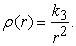(31)

So we obtain, M being the mass of the sphere having its center in O and a radius r: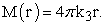(32)

Consequently, v being the velocity of a star at a distance r of O: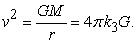(33)

Consequently: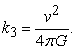(34)

We know also that if ρ0 is the density of the intergalactic ether-substance, then the radius R of the concentration of ether-substance constituting the galaxy is given by the expression: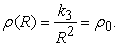(35)

Consequently:(36)

We will call R the “ethered radius” of the considered galaxy.

Let ρ0(5) be the density of the intergalactic medium when the age of the universe was 5 billion years, and ρ0(15) at an age of 15 billion years (presently).

We know that if f is the factor of expansion of the universe between 5 and 15 billion years (we know that f=3, see the PART I of the article, 1st model of the CBE: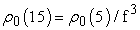(37)

So in a (spiral) galaxy we have 2 different kinds of radius:

The 1st kind of radius, called “ethered radius”, is the radius of the concentration of ether-substance. The 2nd kind of radius is the radius of the disc containing all the stars. We will call “baryonic radius” this second kind of radius. We remark that at a given time, the ethered radius must be greater than the baryonic radius.

We can define rB(15) as the present baryonic radius of the milky way. We know that rB(15) is approximately equal to 50000 years light. If R(15) is the present ethered radius of the milky way, we could assume (because we will see that it leads to results in agreement with observation) that R(15) is approximately 10 times greater than rB(15) (approximately 500000 years light):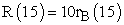(38)

Of course we ignore the real value of R(15), but we will see that our hypothesis gives is in agreement with observations.

Let rB(5) be the baryonic radius of the milky way when the age of the Universe was 5 billion years. Considering that the baryonic radius increases with time, we have the relation: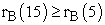(39)

Moreover, using the equation (37), we obtain: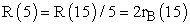(40)

Using the equation (39) and (40) we obtain that at an age of the Universe of 5 billion years, the ethered radius was greater than the baryonic radius: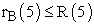(41)

We remark that the previous relation would remain true for a galaxy with the same ethered radius but with a baryonic radius twice greater than the radius of the milky way. (We just take r’B(15) equal to 100000 years light and replace the equation (48) by the equation: R’(15)=5r’B(15)). Our model is valid if we consider that the definitive ethered radius is reached when the Universe was 5 billion year old, or later.

2.4. Thermal Transfer to a Moving Galaxy

We remark that the phenomena of absorption and of emission of ether-substance by a galaxy that we described in the Section 2 (Part II) modifies the thermal equilibrium that we used in the PART I in order to obtain the Tully-Fisher’s law.

We remark that we can consider that the absorption and the emission of ether-substance by a galaxy leads to a thermal transfer defined by a power ε(t) dissipated by the concentration of ether-substance. (Obviously, ε(t) depends on the ethered radius of the moving galaxy, its velocity and the density of the intergalactic ether-substance).

If we make the approximation that ε(t) is negligible compared with the power emitted by the baryons of a galaxy towards the ether-substance, than we can keep the thermal model used to obtain the Tully-Fisher’s law.

2.5. Concentration of Ether-Substance Around Stars and Planets

It is logical to assume that because of gravitation, a concentration of ether-substance occurs around planets and stars.

Let us for instance consider a star with a mass M and a (baryonic) radius RBS. The equation of equilibrium is, for an element of ether-substance with a density ρ(r), a width dr, a surface dS situated at a distance r from O the center of the sun, P(r) being the pressure at this distance of O, supposing r>RBS: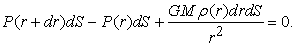(42)

We remind (Equation (2)) that we have P(r)=k1ρ(r) with k1=k0T, T temperature of the concentration of ether-substance.

So we obtain, solving easily the previous differential equation: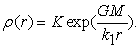(43)

It is possible, but not certain, that as in our model of spiral galaxies, the concentration of ether-substance around the mass M be at a different temperature from the temperature of the galaxies between stars.

It is logical to assume in a first model that if ρ0 is the density of the ether-substance surrounding the concentration of ether-substance linked to the sun, K=ρ0. So we have: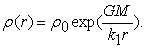(44)

This model leads to incoherencies (ρ(r) too great)

A second possible model is the following one. Let us suppose that RBS is the baryonic radius of the considered star, RES being the radius of the concentration of ether-substance around the star: Then we have RBS=RES, and a concentration of ether-substance on the star calculated with this condition, using the equation of equilibrium for r<RBS.

In a third model (the most probable), there is no concentration of ether-substance on the star. The density ρ(r) is equal to ρ0.

We can apply the same models to galaxies that are satellites of the milky way, as for instance the Large Magellanic cloud .

We remind that we justified that a moving spherical concentration of ether-substance inside the surrounding ether-substance (for instance in the case of a galaxy) kept its mass and was not slowed down nor accelerated. We have a second justification: Let us suppose that the moving spherical concentration of ether-substance expels a little more ether-substance than it absorbs. Let us suppose for instance that the difference is δm. Then the equation of equilibrium remaining the same, we can assume that the spherical concentration of ether-substance will gain also the missing mass δm. Consequently the mass of the concentration of ether-substance remains the same. Moreover δm is absorbed from the surrounding ether-substance. Consequently the balance between the absorbed and the expelled surrounding ether-substance is equal to 0. Consequently, this is a second and more general justification that the spherical concentration of ether-substance is not accelerated nor slowed down.

We remind that in the PART I of our article, we made the hypothesis that the baryons transmitted some energy to the ether-substance surrounding them only if their temperature was superior. It is also possible that baryons transmit some energy to the ether-substance even if their temperature is inferior. We can justify this by the following argument:

We can admit that a baryon vibrates if it is at any temperature (The more it vibrates the higher is its temperature). Then the ether-substance surrounding the baryon slows down this vibration and consequently baryons communicate, whatever be their temperature, an energy to this ether-substance that is converted in thermal energy.

2.6. Conclusion

So we obtained the very interesting result that the motion of a spherical concentration of ether in the intergalactic space does not modify its mass nor its velocity. Moreover we defined 2 different radius for a galaxy, the ethered radius and the baryonic radius. We verified in the case of the milky way that the ethered radius must be greater that the baryonic radius at a given age of the Universe. We also established the evolution as a function of time of the ethered radius. We also made an important approximation in the thermal model permitting to obtain the Tully-Fisher’s law.

### References

  Stacy Mc Gaugh, A Novel Test of Modified Newtonian Dynamics with Gaz rich Galaxies, Physical Review Letter, open archives arXiv.In article  Thierry Delort, Théories d’ or 6e édition, Editions Books on Demand, Paris (2013).In article  T. Delort, Theory of Ether, Physics Essays 13, 4 (2000).In article  T. Delort, Applications of Theory of Ether, Physics Essays 17,3 (2004).In article  D.J Raine,E.G Thomas, An introduction to the science of Cosmology, Institute of physics, London (2001).In article  J.V Narlikar, An introduction to Cosmology, Cambridge University press, Cambridge (2002).In article  A. Aspect, P.Grangier, G.Roger, Experimental realization of Einstein-Podolsky-Rosen-Bohm-Gedanken experiment: A new violation of Bell’s inequalities, Phys.Rev.letter (1982).In article  G. A Tammann and B. Reindl, Astronomy and Astrophysics 549(2013) (on arXiv).In article  P. Kroupa, M. Pawlowski, M. Milgrom, The failures of the standard model of Cosmology require a new paradigm, International Journal of Physics D21 (2012).In article  M. Milgrom, A modification of the Newtonian dynamics as a possible alternative to the hidden mass hypothesis, Astrophysical Journal 270 (1983).In article  Perlmutter et al, Discovery of a supernova explosion at half the age of the universe, Nature 391, 51-54 (1998).In article  D. R Alves, C. A Nelson, The rotation curve of the Large Magellanic cloud and the implications for Microlensing, The astrophysical journal (October 2000).In article  T. Delort, Ether,dark matter and topology of the Universe, open archives vixra, Internet archives.In article